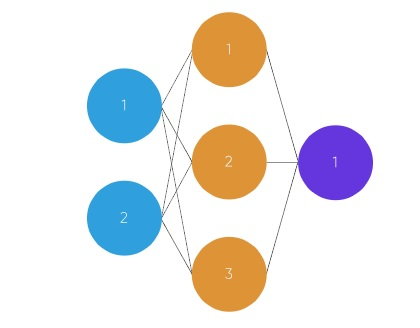# A single neuron neural network in Python

PythonProgrammingServer Side Programming

Neural networks are very important core of deep learning; it has many practical applications in many different areas. Now a days these networks are used for image classification, speech recognition, object detection etc.

Let’s do understand what is this and how does it work?

This network has different components. They are as follows −

• An input layer, x
• An arbitrary amount of hidden layers
• An output layer, ŷ
• A set of weights and biases between each layer which is defined by W and b
• Next is a choice of activation function for each hidden layer, σ.

In this diagram 2-layer Neural Network is presented (the input layer is typically excluded when counting the number of layers in a Neural Network)In this graph, circles are representing neurons and the lines are representing synapses. The synapses are used to multiply the inputs and weights. We think weights as the “strength” of the connection between neurons. Weights define the output of a neural network.

Here’s a brief overview of how a simple feed forward neural network works −

When we use feed forward neural network, we have to follow some steps.

• First take input as a matrix (2D array of numbers)

• Next is multiplies the input by a set weights.

• Next applies an activation function.

• Return an output.

• Next error is calculated, it is the difference between desired output from the data and the predicted output.

• And the weights are little bit changes according to the error.

• To train, this process is repeated 1,000+ times and the more data is trained upon, the more accurate our outputs will be.

HOURS STUDIED, HOURS SLEPT (INPUT)TEST SCORE (OUTPUT)

2, 992
1, 586
3, 689
4, 8?

## Example Code

from numpy import exp, array, random, dot, tanh
class my_network():
def __init__(self):
random.seed(1)
# 3x1 Weight matrix
self.weight_matrix = 2 * random.random((3, 1)) - 1
defmy_tanh(self, x):
return tanh(x)
defmy_tanh_derivative(self, x):
return 1.0 - tanh(x) ** 2
# forward propagation
defmy_forward_propagation(self, inputs):
return self.my_tanh(dot(inputs, self.weight_matrix))
# training the neural network.
deftrain(self, train_inputs, train_outputs,
num_train_iterations):
for iteration in range(num_train_iterations):
output = self.my_forward_propagation(train_inputs)
# Calculate the error in the output.
error = train_outputs - output
adjustment = dot(train_inputs.T, error *self.my_tanh_derivative(output))
# Adjust the weight matrix
self.weight_matrix += adjustment
# Driver Code
if __name__ == "__main__":
my_neural = my_network()
print ('Random weights when training has started')
print (my_neural.weight_matrix)
train_inputs = array([[0, 0, 1], [1, 1, 1], [1, 0, 1], [0, 1, 1]])
train_outputs = array([[0, 1, 1, 0]]).T
my_neural.train(train_inputs, train_outputs, 10000)
print ('Displaying new weights after training')
print (my_neural.weight_matrix)
# Test the neural network with a new situation.
print ("Testing network on new examples ->")
print (my_neural.my_forward_propagation(array([1, 0, 0])))

## Output

Random weights when training has started
[[-0.16595599]
[ 0.44064899]
[-0.99977125]]
Displaying new weights after training
[[5.39428067]
[0.19482422]
[0.34317086]]
Testing network on new examples ->
[0.99995873]
Advertisements